Courses

# GATE Past Year Questions: Forced Vibration Notes | EduRev

## Mechanical Engineering : GATE Past Year Questions: Forced Vibration Notes | EduRev

The document GATE Past Year Questions: Forced Vibration Notes | EduRev is a part of the Mechanical Engineering Course Theory of Machines (TOM).
All you need of Mechanical Engineering at this link: Mechanical Engineering

Try yourself:The equation of motion for a spring-mass system excited by a harmonic force is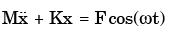where M is the mass, K is the spring stiffness, F is the force amplitude and w is the angular frequency of excitation. Resonance occurs when w is equal to



Try yourself:The damping ratio for a viscously damped spring mass system, governed by the relationship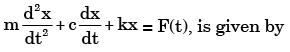Try yourself:A solid disc with radius a is connected to a spring at a point d above the center of the disc.
The other end of the spring is fixed to the vertical wall. The disc is free to roll without slipping on the ground. The mass of the disc is M and the spring constant is K. The polar moment of inertia for the disc about its centre
is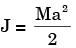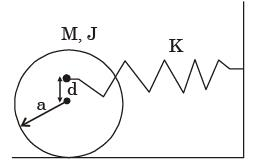The natural frequency of this system in rad/s is given by



Try yourself:A single degree of freedom mass-spring-viscous damper system with mass m, spring constant k and viscous damping coefficient q is critically damped. The correct relation among m, k, and q is



Try yourself:Which of the following statements are TRUE for damped vibrations?
P. For a system having critical damping, the value of damping ratio is unity and system does not undergo a vibratory motion.
Q. Logarithmic decrement method is used to determine the amount of damping in a physical system.
R. In case of damping due to dry friction between moving surfaces resisting force of constant magnitude acts opposite to the relative motion.
S. For the case of viscous damping, drag force is directly proportional to the square of relative velocity.



Try yourself:Consider a single degree of freedom system with viscous damping excited by a harmonic force. At resonance, the phase angle (in degree) of the displacement with respect to the exciting force is



Try yourself:Critical damping is the



Try yourself:A mass of 1 kg is attached to two identical springs each with stiffness k = 20 kN/m as shown in the figure. Under frictionless condition, the natural frequency of the system in Hz is close to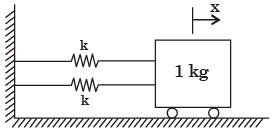Try yourself:A mass m attached to a spring is subjected to a harmonic force as shown in figure. The amplitude of the forced motion is observed to be 50 mm. The value of m (in kg) is​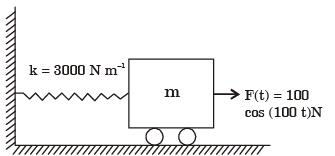Try yourself:A vehicle suspension system consists of a spring and a damper. The stiffness of the spring is 3.6 kN/m and the damping constant of the damper is 400 Ns/m. If the mass is 50 kg, then the damping factor (d) and damped natural frequency (fn), respectively, are



Try yourself:An automotive engine weighing 240 kg is supported on four springs with linear characteristics. Each of the front two springs have a stiffness of 16 MN/m while the stiffness of each rear spring is 32 MN/m. The engine speed (in rpm), at which resonance is likely to occur, is



Try yourself:A vibratory system consists of a mass 12.5 kg, a spring of stiffness 1000 N/m, and a dashpot with damping coefficient of 15 Ns/m.
The value of critical damping of the system is



Try yourself:The differential equation governing the vibrating system is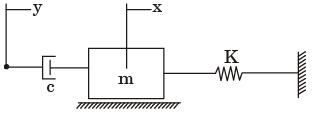Try yourself:In a spring-mass system, the mass is 0.1 kg and the stiffness of the spring is 1 kN/m. By introducing a damper, the frequency of oscillation is found to be 90% of the original value. What is the damping coefficient of the damper?



Try yourself:There are four samples P, Q, R and S with natural frequencies 64,96,128 and 256 Hz, respectively. They are mounted on test setups for conducting vibration experiments. If a loud pure note of frequency 144 Hz is produced by some instrument, which of the samples will show the most perceptible induced vibration?



Try yourself:A mass m, of 20 kg is attached to the free end of a steel cantilever beam of length 1000 mm having a cross-section of 25 × 25 mm. Assume the mass of the cantilever to be negligible and Esteel = 200 GPa. If the lateral vibration of this system is critically damped using a viscous damper, the damping constant of the damper is​



Try yourself:A uniform rigid slender bar of mass 10 kg, hinged at the left end is suspended with the help of spring and damper arrangement as shown in the figure where K= 2 kN/m, C = 500 Ns/m and the stiffness of the torsional spring kq is 1 kN /m/rad. Ignore the hinge dimensions.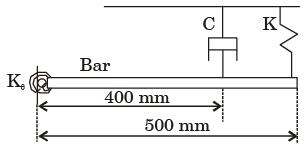The damping coefficient in the vibration equation is given by



Try yourself:A uniform rigid slender bar of mass 10 kg, hinged at the left end is suspended with the help of spring and damper arrangement as shown in the figure where K= 2 kN/m, C = 500 Ns/m and the stiffness of the torsional spring kq is 1 kN /m/rad. Ignore the hinge dimensions.​The undamped natural frequency of oscillations of the bar about the hinge point is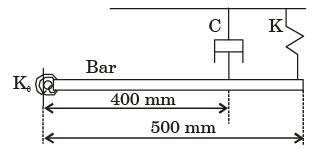Offer running on EduRev: Apply code STAYHOME200 to get INR 200 off on our premium plan EduRev Infinity!

## Theory of Machines (TOM)

94 videos|41 docs|28 tests

,

,

,

,

,

,

,

,

,

,

,

,

,

,

,

,

,

,

,

,

,

;